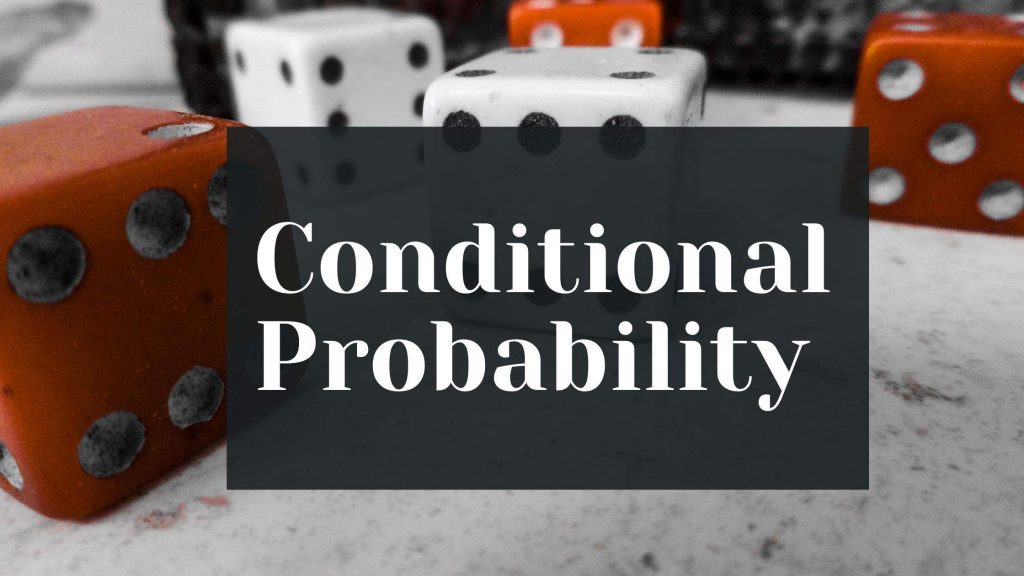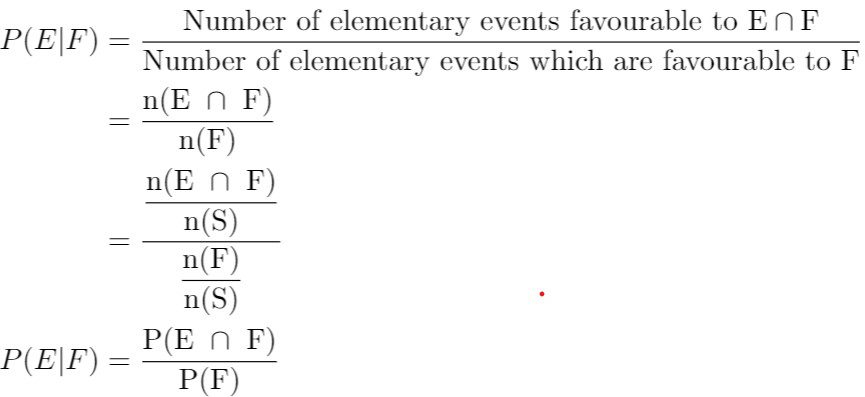Maths
Study Material

# Conditional Probability and Its Properties

This blog discusses how conditional probability is defined and also in what ways it is useful. Few solved problems are presented for better understanding of the viewer.

2 minutes longHesap Oluştur

Got stuck on homework? Get your step-by-step solutions from real tutors in minutes! 24/7. Unlimited.

Conditional probability deals with the questions/paradigms in which we have to find how the occurrence of an event affects another event. Machine Learning and Artificial Intelligence, and many statistically related computer science, have conditional probability as their roots.

## Conditional probability

The conditional probability of E given that F has occurred, denoted by P(E|F), can be calculated as P(E ∩ F) / P(F).A necessary condition is P(F) ≠ 0 i.e., F ≠ Φ.

## Properties of conditional probability

Let E and F are two events of a sample space S of an experiment,

Property 1:
P(S|F) = P(F|F) = 1, which can be easily derived using the relation S ∩ F = F and F ∩ F = F.
Property 2:
If A and B are two events of a sample space S and F is an event of S such that P(F) ≠ 0, then
P((A ∩ B)|F) = P(A|F) + P(B|F) – P((A ∩ B)|F).
Property 3:
P(E’|F) = 1 – P(E|F)

## Solved Problems

### For Example 1

A family has two children. What is the probability that both the children are girls given that at least one of them is a girl?
Solution:
Let us define two events E and F,
E : ‘both the children are girls’
F : ‘at least one of the child is a girl’
E = {GG}, F = {GB, BG, GG}
P(E|F) = P(E ∩ F)/ P(F) = n(E ∩ F)/ n(F) = 1/3,
Note: we could have calculated P(E ∩ F) and P(F) using S = {BB, GB, BG, GG}
then P(E ∩ F) = n(E ∩ F)/n(S) = 1/4 and P(F) = n(F)/n(S) = 3/4
P(E|F) = P(E ∩ F)/ P(F) = (1/4)/(3/4) = 1/3.

### For Example 2:

Given that E and F are events such that P(E) = 0.5, P(F) = 0.4 and P(E ∩ F) = 0.2, find P(E|F) and P(F|E)?
Solution:
We know that P(E|F) = P(E ∩ F)/ P(F), P(E|F) = 0.2/0.4 = 1/2 = 0.5
and changing the variables in the above formula, we get
P(F|E) = P(F ∩ E)/ P(E) = 0.2/ 0.5 = 2/5 = 0.4